#DAY 24
0

## RNA-Sick@Day24 > 關於資料視覺化，我想說的是｜用 python 繪製充滿特色的圖表 feat. seaborn

### 用 seaborn 繪製特色圖表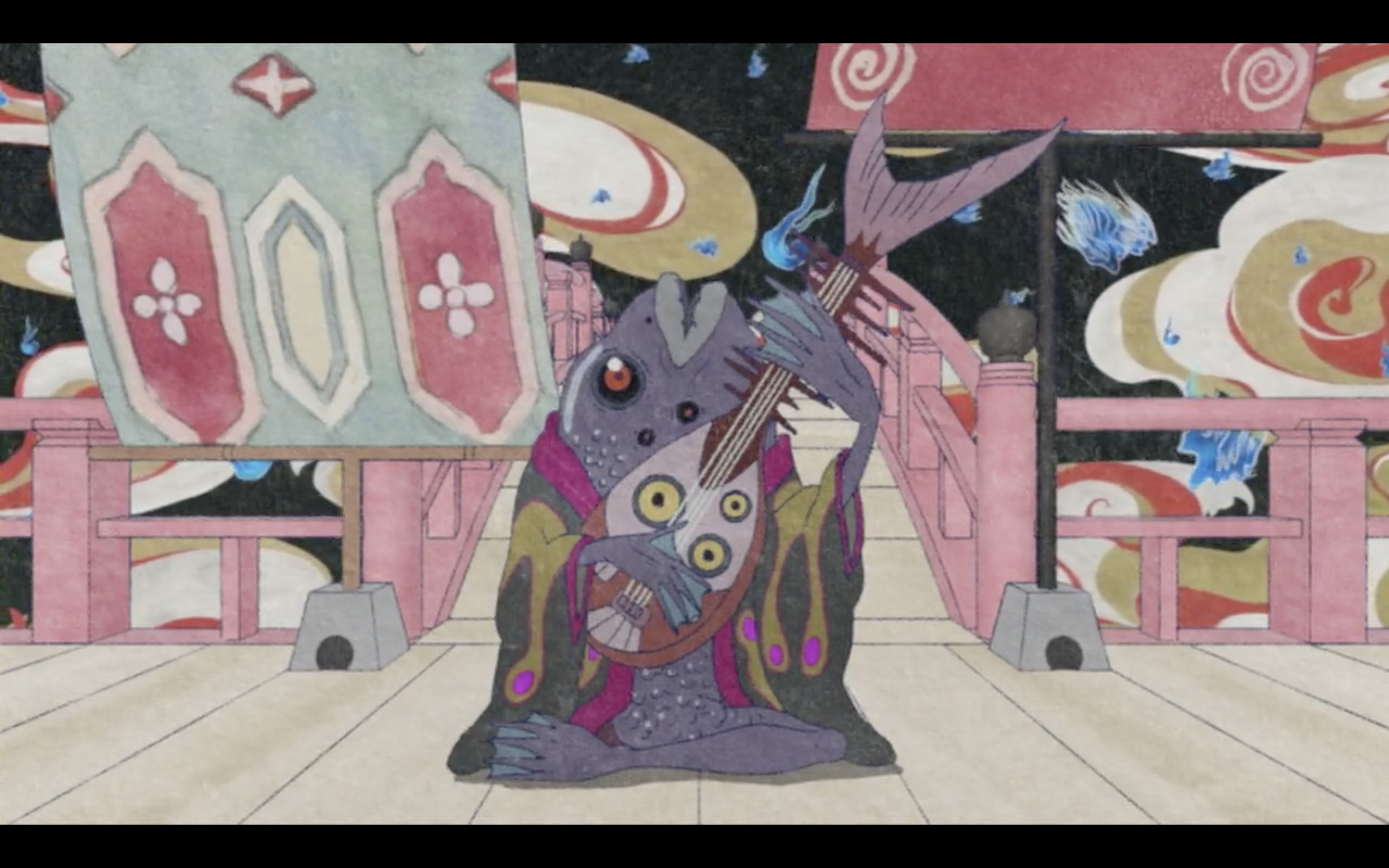(截圖來自怪化貓海坊主)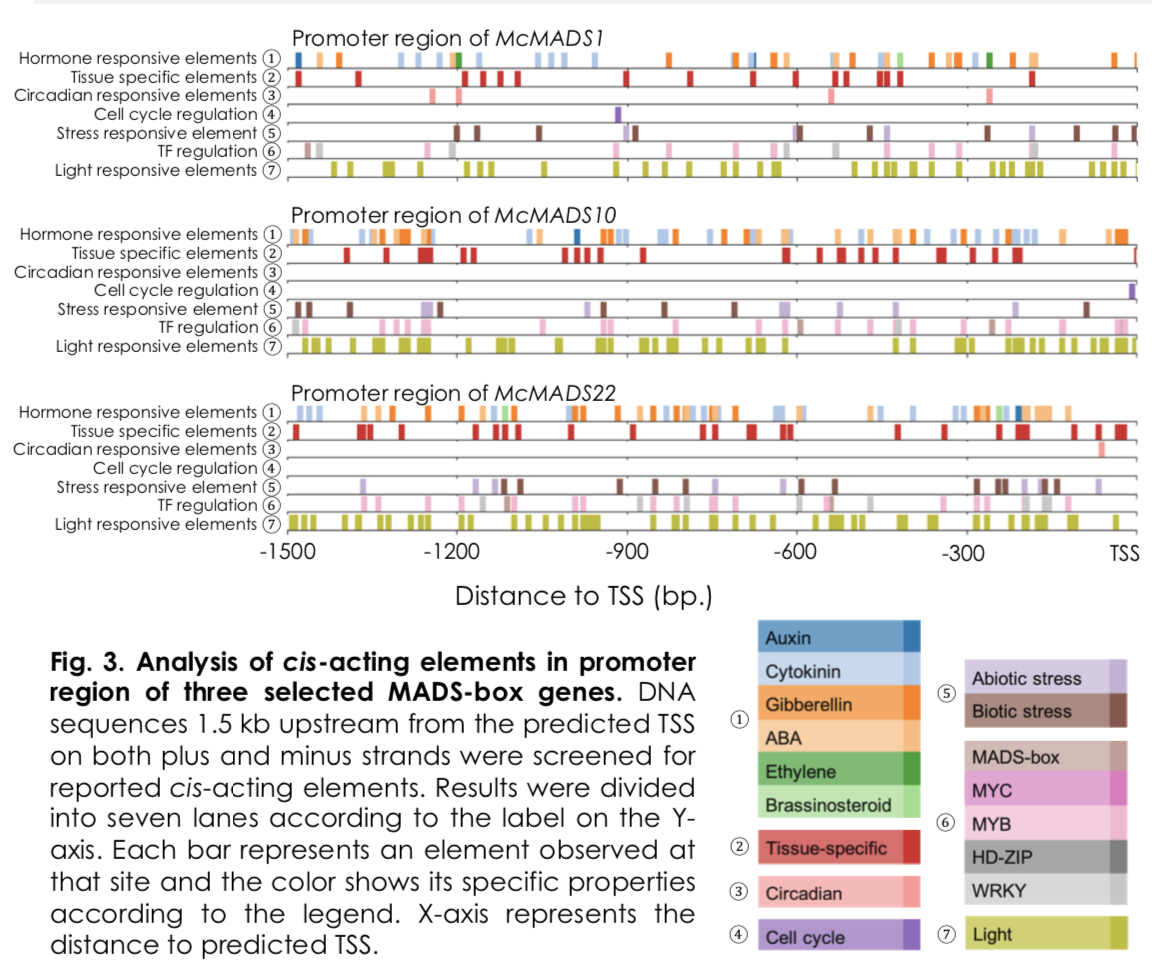(謝晨、杜宜殷. 2019. Genome-wide Identification and Analysis of MADS-box Gene Family in Bitter Gourd.)

### 準備所需資料環節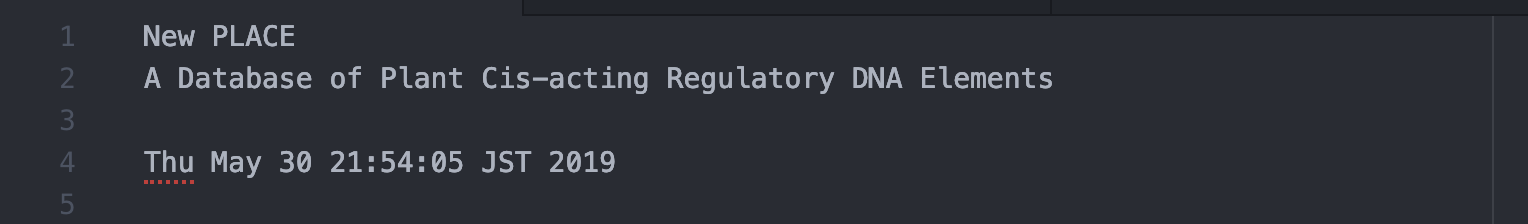fasta 格式序列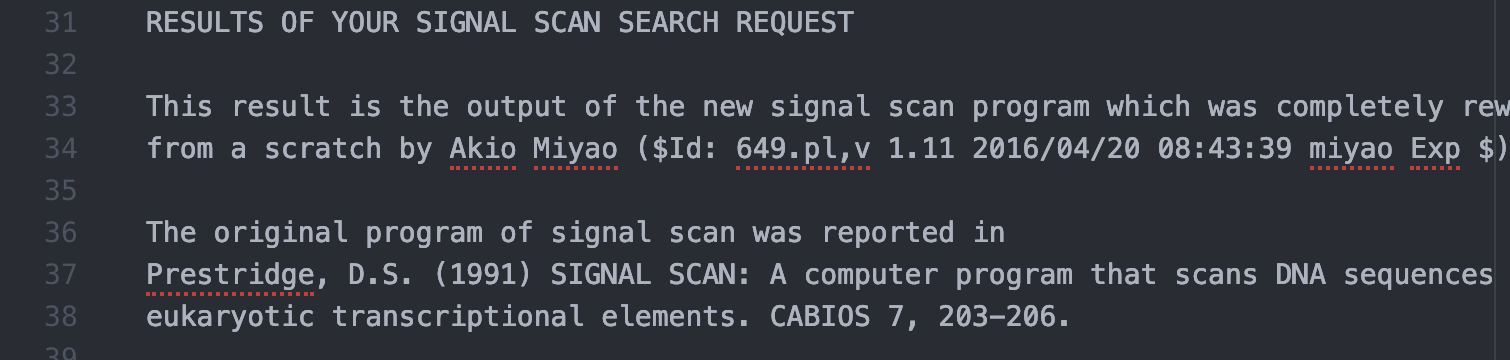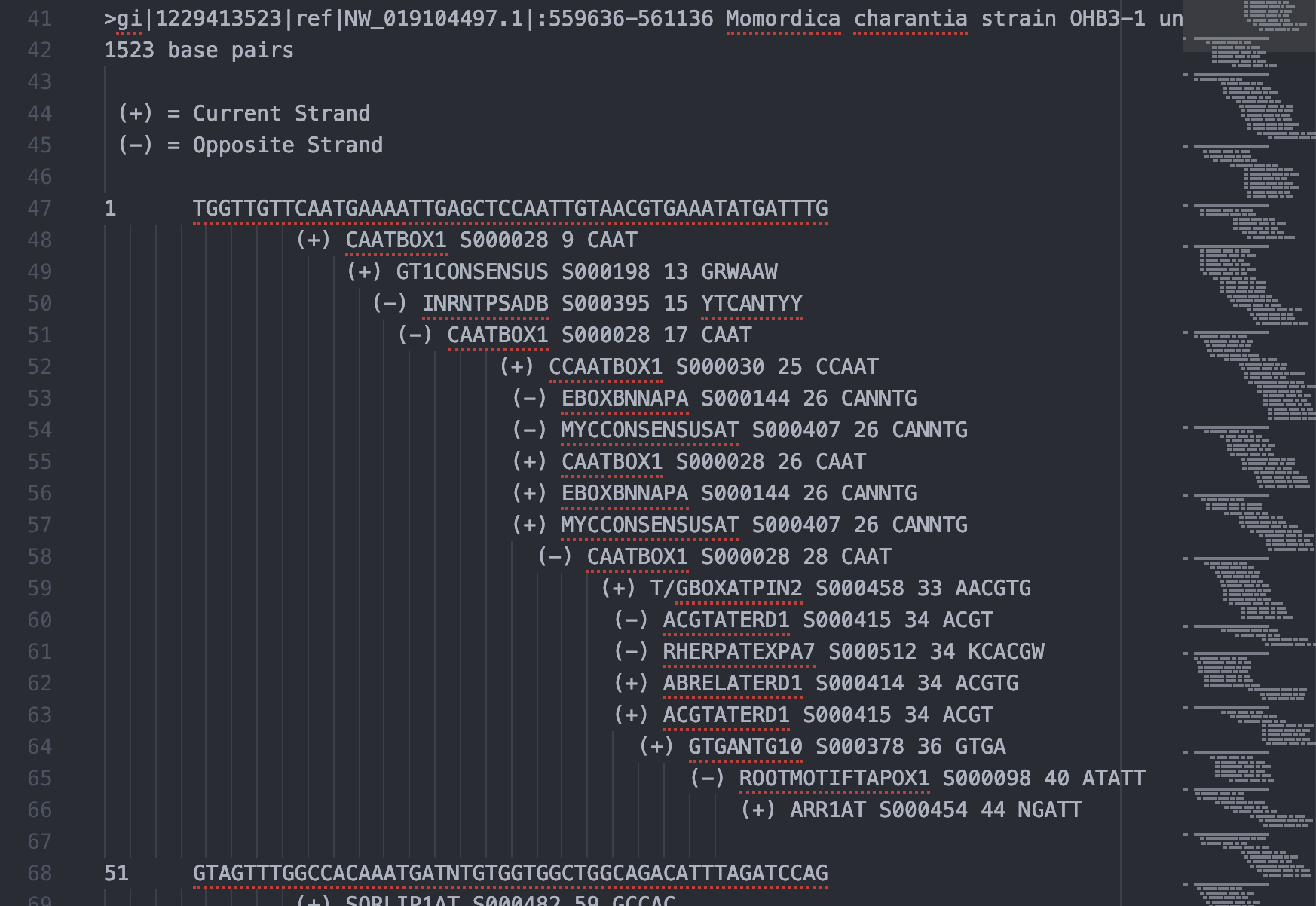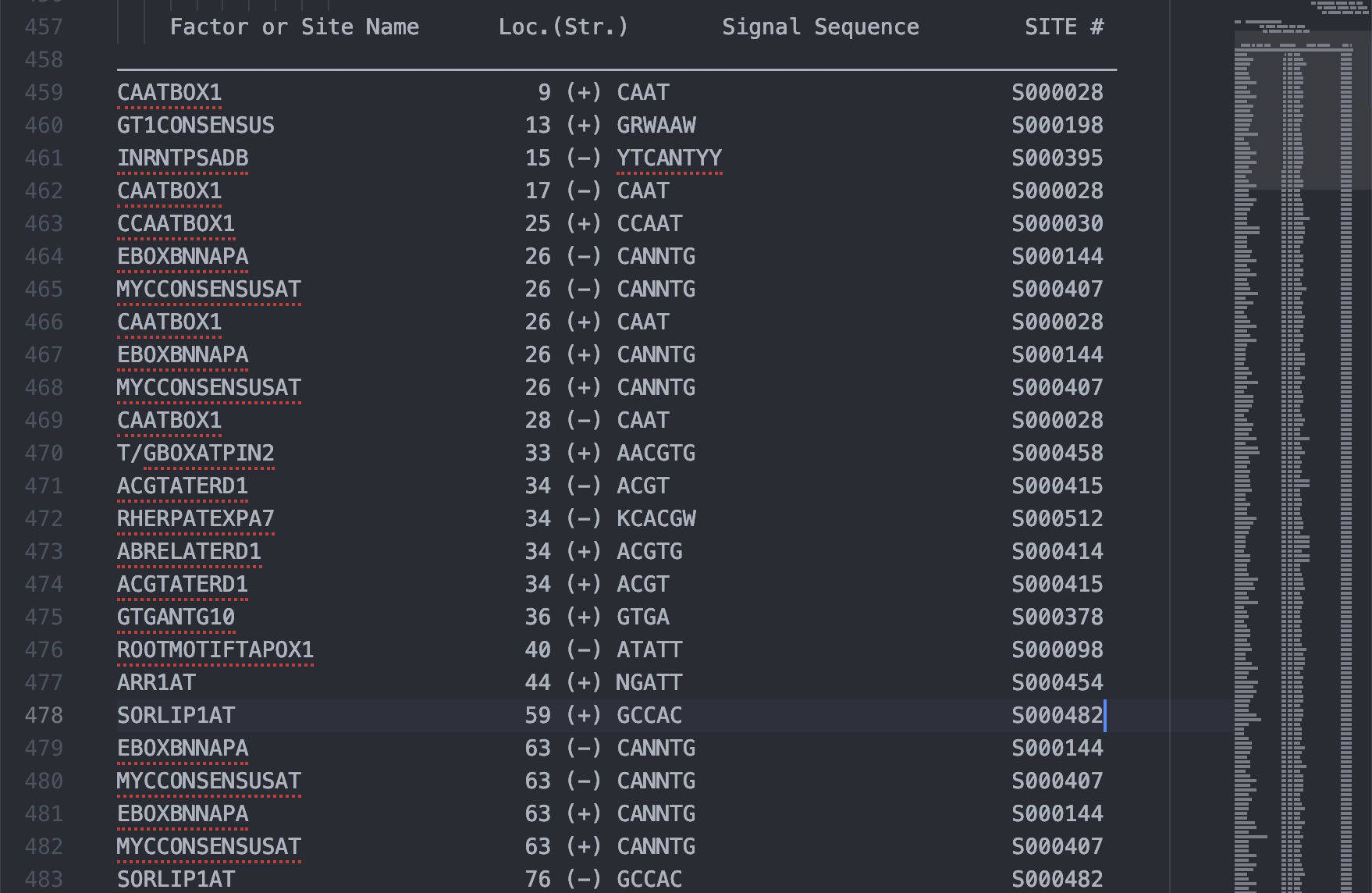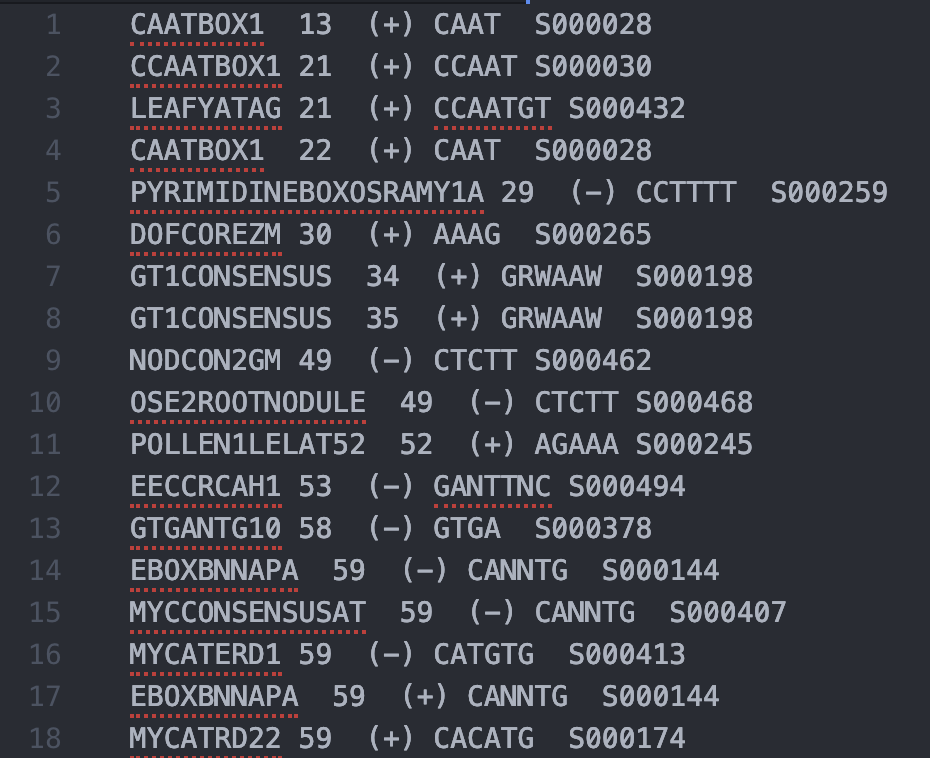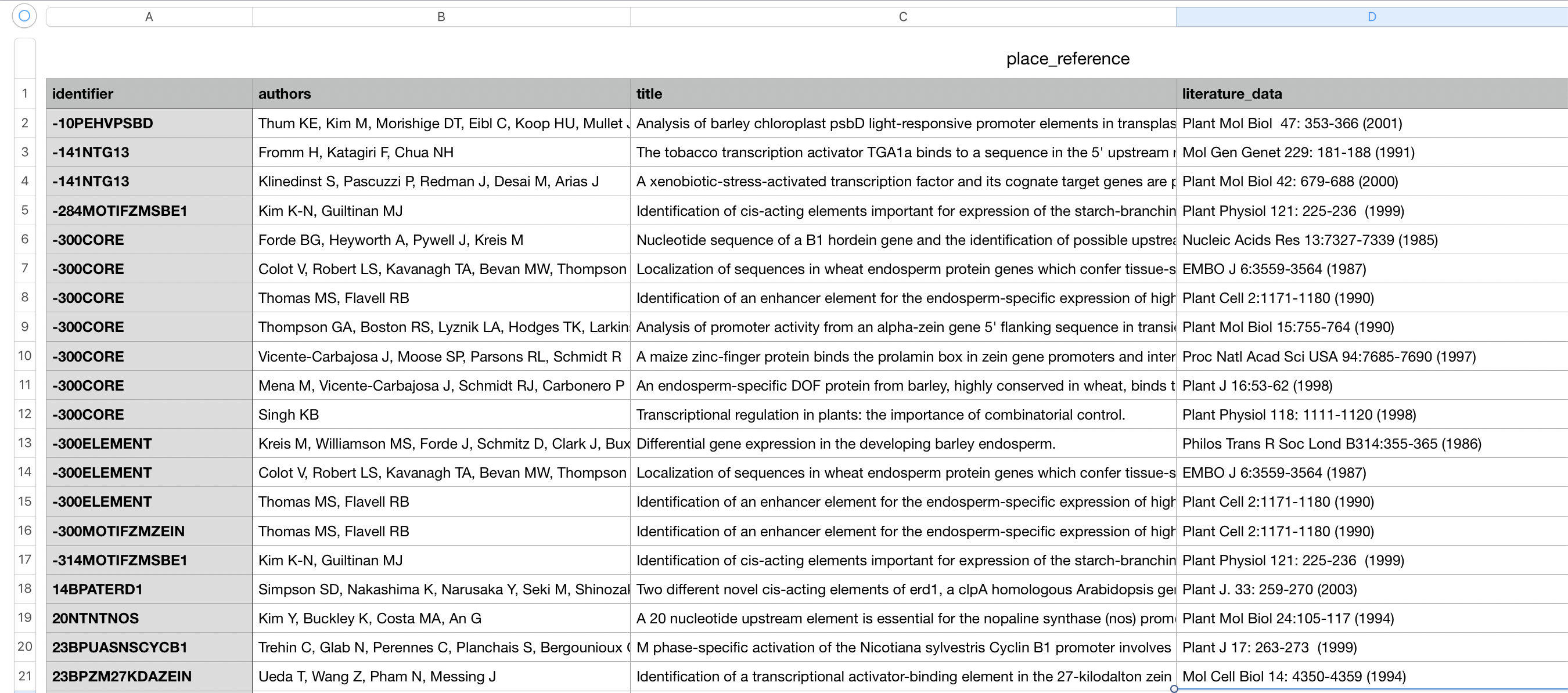### 資料讀取與基本參數設定環節

``````import csv
import pandas as pd
import numpy as np
import seaborn as sns
import matplotlib.pyplot as plt
import matplotlib
%matplotlib inline

promoter = promoter.set_index(0)
``````

### 順式作用元件與繪圖參數設定環節

PLACE 的參考資料表中，有著該段順式作用元件的代號、DNA 序列內容、發表文獻標題。從發表文獻之標題就可以了解該順式作用元件的功能。因此我準備了一個雙層的 list 來存放我認為夠有辨識度的關鍵字，第一層 list 用來存放不同的屬性之關鍵字，比如同樣屬於植物賀爾蒙的就放在第一個 list 組成中，跟組織部位有關的則放在第二個組成中；第二層則用以存放相同屬性的同義詞，比如同樣跟植物生長素有關的元件之文獻標題可能是 auxin 或 SAUR。這將在實際繪圖階段透過迴圈來逐個存取並辨識歸類。

``````term_list =
[
[
["auxin","Auxin","SAUR"],
["cytokinin","Cytokinin"],
["gibberellin","Gibberellin","GA"],
["ABA","abscisic acid","abscisic acid"],
["ethylene","ETHYLENE", "Ethylene"],
["brassinosteroid"]
],

[
["tissue"]
],

[
],

[
["cell cycle","Cell cycle","phase-","E2F"]
],

[
["abiotic stress","CBF","heat","cold", "salt","low-temp"],
["biotic stress","infected"]
],

[
["MYC"],
["MYB"],
["HD-ZIP"],
["WRKY"]
],

[
["light","Light"]
]
]

fig, ax = plt.subplots(len(term_list),1,figsize=(15, len(term_list)*0.3))
cmap = matplotlib.cm.get_cmap('tab20')
xtick = range(0, 1501, 300)
color_ch = 0.04
ih = 1
ax_ch = 0
lw = 6
plt.rcParams.update({'font.size': 29})
``````

### 繪圖環節

``````ax_ch = 0
for term_class in term_list:
ax[ax_ch].set_xlim(0,1500)
ax[ax_ch].set_ylim(0,1)
ax[ax_ch].spines['left'].set_visible(False)
ax[ax_ch].spines['top'].set_visible(False)
ax[ax_ch].spines['right'].set_visible(False)
ax[ax_ch].get_yaxis().set_visible(False)
ax[ax_ch].set_xticklabels([])
ax[ax_ch].set_xticks(xtick)
for term_subclass in term_class:
title_list = []
id_list = []
for term in term_subclass:
for i in range(place.shape):
title = place.loc[i]["title"]
if (term in str(title)):
id = place.loc[i]['identifier']
id_list.append(id)
title_list.append(title)
id_list = list(set(id_list))
id_filter = promoter.index.isin(id_list)
x = promoter[id_filter]
rgba = cmap(color_ch)
vv = list(x.values)
vv = list(set(vv))
sns.rugplot(vv, color = rgba, height = ih, ax = ax[ax_ch], linewidth = lw)
color_ch+=0.05
ax_ch +=1
fig
``````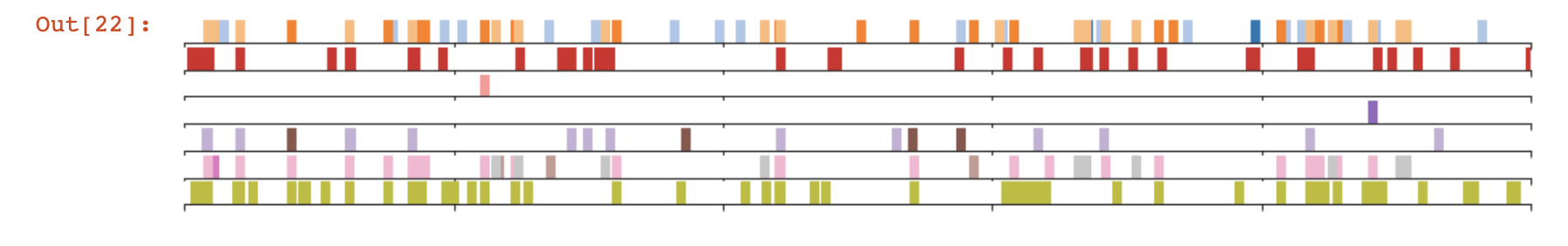### 參考資料與延伸閱讀

An introduction to seaborn - seaborn 0.9.0 documentation

PLACE - Reference